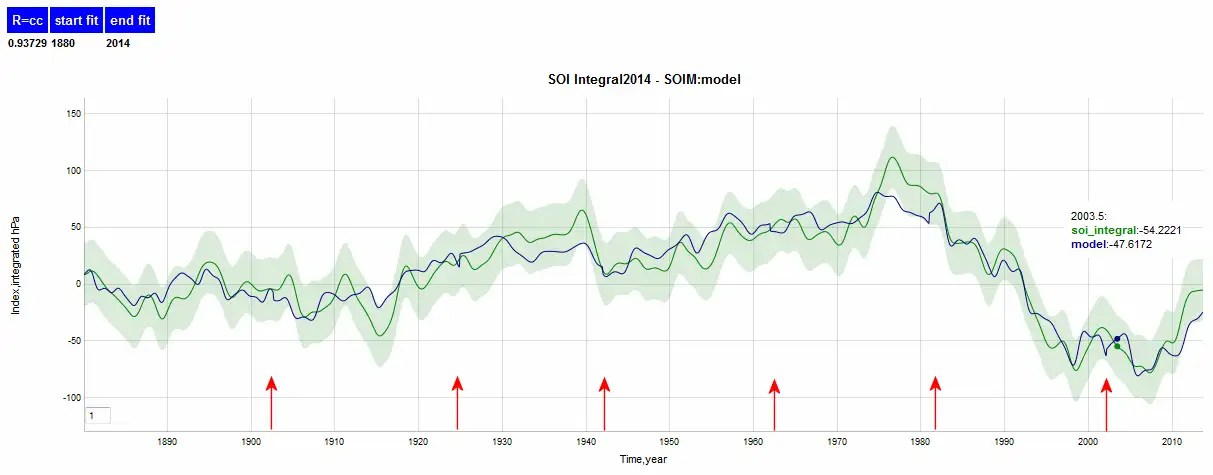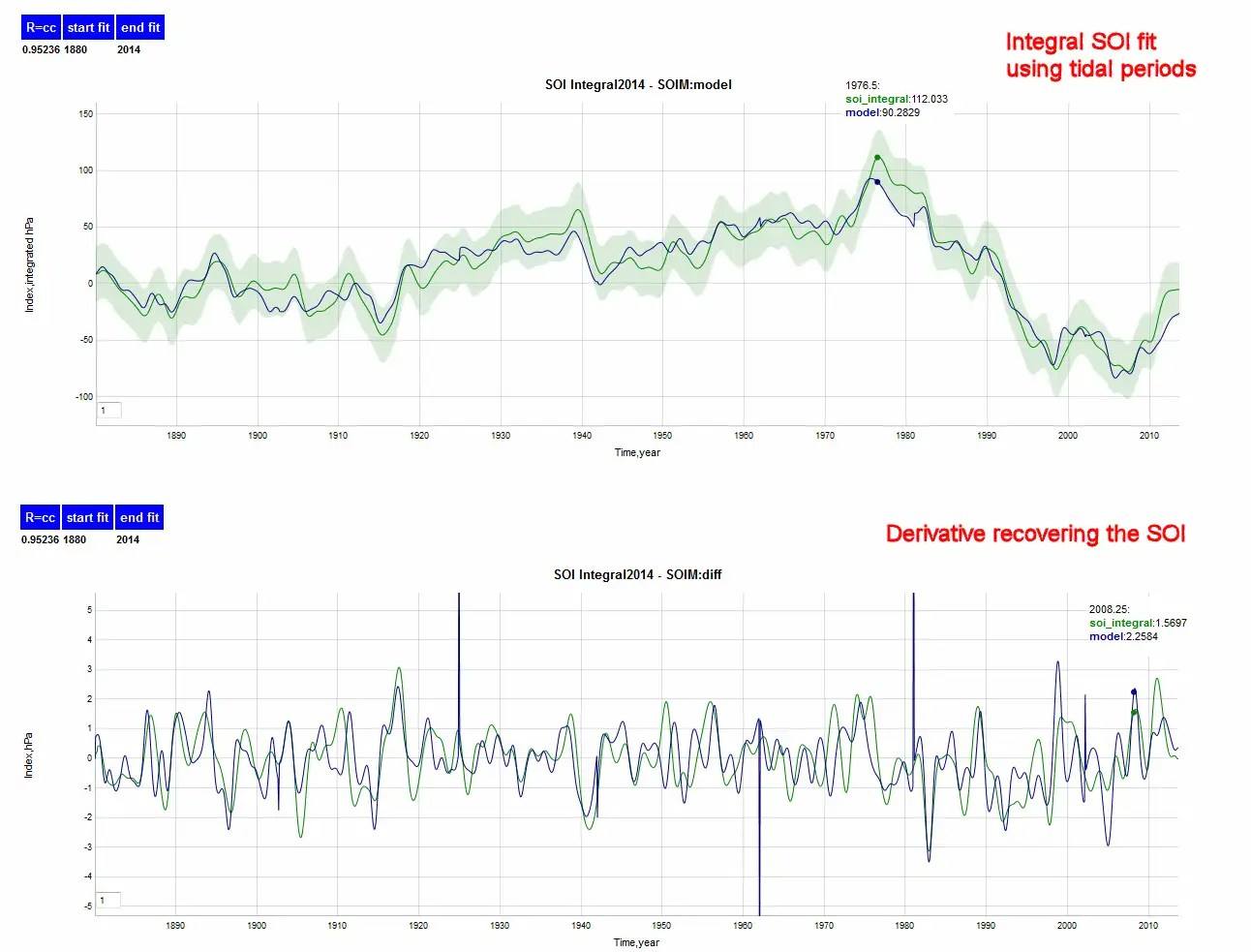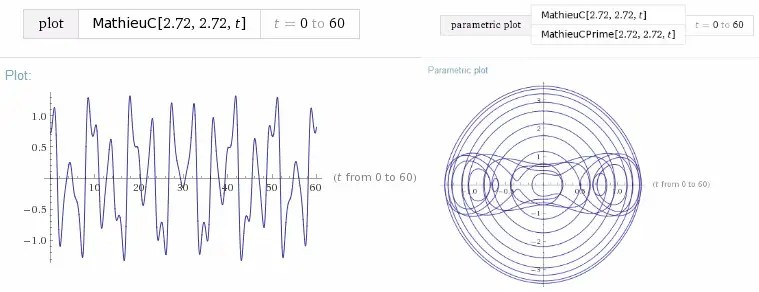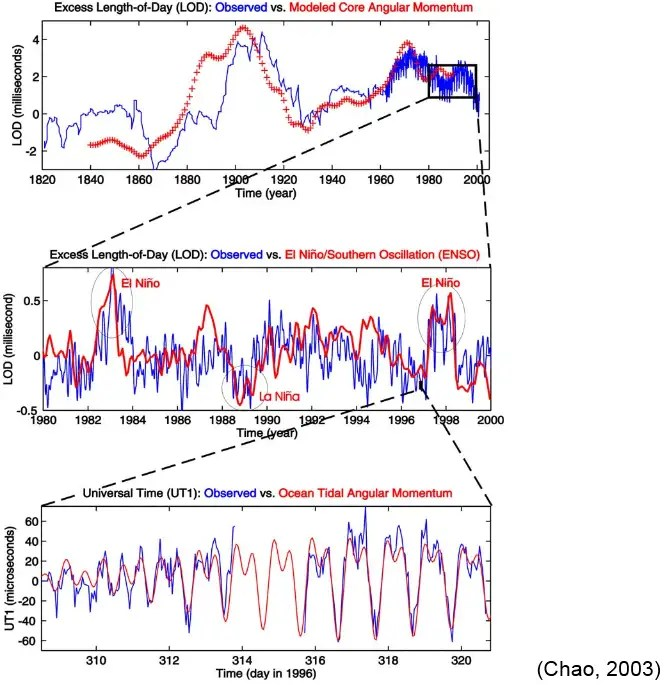# The Chandler Wobble and the SOIM

(see later posts here)

[mathjax]Seth Carlo Chandler Jr was an actuary who studied his namesake wobble for thirty years.

ENSO and the Southern Oscillation Index has confounded everyone with its unpredictability.

Could a connection exist between the ENSO and the Chandler Wobble ? 

Based on what I have been analyzing with respect to the ENSO data, I am leaning in that direction.  In the first post on the Southern Oscillation Index Model (SOIM), my initial analysis lead to a fundamental Mathieu frequency T of 6.3 years, a value of a = 2.83  and q = 2.72 :

I followed that up with additional checks and analogies to other physical phenomena :

That culminated with a trial fit of the SOI with a set of Mathieu parameters. Yet — even though the fit was decent — I was not satisfied with the result as it tended to overfit with respect to the adjustable parameters.  The ideal situation would limit the number of fundamental frequency terms.

Three observations lead me to a much simplified representation.

• The main Mathieu frequency of 6.3 years seemed to vary over the historical record.
• The pressure index of the SOI is essentially a differential measure, and so the derivative of the Mathieu function should be fit to the pressure, e.g. use MathieuCPrime and not MathieuC .
• The connection between the original fit of 6.3 years and the Chandler Wobble beat frequency of 6.39 years (= 1/(1-365.25/433)), and the fact that this measure has been known to vary over the past 100+ years.

↓ ↓ ↓ ↓ ↓ ↓ ↓ ↓ ↓ ↓ ↓ ↓ ↓ ↓ ↓ ↓ ↓ ↓ ↓ ↓

The excuse for the overfitting I believe was due to the slight variation of the characteristic Mathieu frequencies over the 130+ year time span of the SOI. When fit over smaller intervals, the convergence turned out much better with far fewer frequency components. However, unless a pattern is found for such a variation in frequencies, it makes it difficult to use for projections. So if we can pinpoint the origin of the fundamental frequency and its variability, we can start  from there.

The first step I took was to use a brute force approach to deduce the characteristic Mathieu frequency.   We start with the Mathieu equation from the first post:

$$frac{d^2Phi}{deta^2}+[a-2qcos(2eta)]Phi=0$$

where

$$eta = omega t$$

Then if we plot the ratio of the SOI index measurement and its second derivative, we can conceivably extract the varying periodic factor from the graphed profile:

$$-frac{frac{d^2Phi}{deta^2}}{Phi} = a-2qcos(2eta)$$

If we make the assumption that dΦ/dt is the SOI index, that means we can use the first derivative of the SOI and its integral to find any periodic values. The tricky part is that the integral wanders away from zero, so I filtered out the low frequency modulation, leaving behind only the subdecadal fluctuation. Although a bit messy with singularities, since the denominator passes through zero as the index wanders through inflection points, the fundamental frequency is clearly discernible, see Figure 1:Figure 1: Plot of the ratio of the integrated index to its first derivative. This will show a periodic function if the SOI follows a Mathieu-type equation. The dotted red line is a 6.35 year periodic function with harmonics of 12.7 years and 6.35/2 years. The sharp vertical lines are caused by singularities in the quotient.  Variations in the periodicities are seen in the spans 1940..1960 where it is ~10% larger and 1880..1900 where it is ~10% smaller.

The important point to note is that a sinusoidal component is clearly present, suggesting that this process does indeed reflect a nonlinear Mathieu equation, and that the main frequency definitely moves around, varying from double periods of 12.7 years paired with weaker indications of a 6.35 year period.

Now if we model the SOI data via piece-wise intervals over the range using only the low-frequency terms (44.1 years and 146.5 years) and the slightly varying 6.35 year fundamental frequency, we get Figure 2 :Fig 2 : Mathieu fit to the integrated SOI model. The up red arrows indicate transition regions for the fundamental 6.35 year frequency. Slight discontinuities in the profile are observed at these points. To model the long term variation, periods of 44.1 years and 146.5 were incorporated into the model.

Figure 3 is the differentiated form, which maps directly to the SOI. Though not close to perfect, enough correlation exists to strong SOI extremes (see 1983) to indicate that this is on the right track.Fig 3 : Mathieu fit to the integrated SOI model when differentiated. The spikes indicate transition regions for the fundamental 6.35 year frequency. Singularities in the differentiation of the integrated profile are observed at these points.

The agreement is good enough that it becomes tempting to add in a few extra fixed periods arising from fundamental tidal beat frequencies of 6 years, 8.85 years, 18.6, and 16.9 years, corresponding to various combinations of the draconic, sidereal, and anomalistic lunar months , see Figure 4.  In particular, Royer  has detected  the 16.9 year period in air temperatures.Fig 4:  Explanation of main lunar beat frequencies, from ref 

Adding these cycles as Mathieu components, we get Figure 5.Fig 5 : Adding the lunar tidal periods of 6, 8.85, 18.6, and 16.9 years tentatively improves the fit.

At this point it is useful to understand how I came to apply the derivative of the Mathieu function, which is really the breakthrough in the analysis. Consider that the parametric plot of the result and it’s first derivative is very useful as a means to characterize a possible solution, as I showed in the initial post. Yet, I didn’t look closely enough at the phase relation, as one see the following figures.  First consider  Figure 6 and Figure 7 which are the index and the phase plot of the actual data.

This is interesting in that the “binocular” effect in the phase plot of Figure 7  matches better the first and second derivative of the Mathieu function (Figure 8), rather than the main and its first derivative (Figure 9) shown below.Figure 8:  Both the first-derivative version of the Mathieu function (MathieuCPrime and MathieuSPrime in Mathematica) and the phase plot of first and second derivatives qualitatively match the observations.

Compare the above against the plain Mathieu phase plot in Figure 9.  The difference is primarily in how the lissajous figure traces out in the vertical versus horizontal directions.Fig 9 :  Plain Mathieu function and its phase plot.

The appendix is a rundown of some other Mathieu-like formulations that I looked at.  The last one is important in that it shows the phase plot of a red noise model, created using an Ornstein-Uhlenbeck process.  Some climate scientists propose that the variability in the Pacific ocean indices is close to red noise .  This is plausible , as one model of an O-U process is of a random walker oscillating back-and-forth within a potential well.

Yet, the evidence points to the even more plausible explanation of a limited set of periodic or quasi-periodic factors interacting with a nonlinear wave equation (i.e. Mathieu equation) to produce the fluctuating pattern in the SOI profile.

## Discussion

This brings us to the Chandler wobble connection. As Gross suggested , the wobble itself is a result of a quasi-periodic oceanic disturbance, and so as a premise we somehow attribute it as a strong factor in the ENSO SOI evolution.

“Using calculations based on applying numerical models of the ocean to data obtained on the Chandler wobble between 1985-95, Dr Gross has determined the principles causes of the wobble. He found the wobble is due to fluctuating pressure on the bottom of the ocean, caused by temperature and salinity changes, and wind-driven changes in the circulation of the oceans.

Like a top wobbling as it spins – only much much slower – the Chandler wobble takes 433 days or 1.2 years to complete one cycle. The amplitude of the wobble amounts to about 6.5 metres at the North Pole. But like a top, eventually the wobble should stop. This is what has puzzled scientists, who have calculated that the Chandler wobble would have stopped in just 68 years, unless some force was acting to keep it going.

Dr Gross calculated that two thirds of the Chandler wobble is caused by changes in pressure at the ocean bottom, and the rest by fluctuations in atmospheric pressure. However, Dr Gross calculated that atmospheric winds and ocean currents had only a minor effect on the wobble. ” [link]

The pole tide caused by the Chandler wobble is only a few millimeters in height, so is not the cause of the effect but a side-effect of the deeper water activity.

The variations in the Chandler wobble have long been known and are best understood by a combination of a naturally damped sharp resonance (with a Q factor over 100) together with a semi-regular forcing factor which restarts the Chandler wobble at intervals of around 20 years, see Figure 10.Fig 10 : The Chandler Wobble envelope is a beat frequency of the annual wobble and the 433 day wobble associated with the shape of the earth. The periods in the above profile change in value and phase at various time. For example, around 1925, the sign of the wobble apparently inverts.

Before becoming too speculative, it is important to remember the strong  fundamental correlations between changes in angular momentum of the earth’s rotation and with oceanic and atmospheric fluctuations. Figure 11 below from Chao  shows how the LOD relates to ENSO, wind variations, and ocean tidal variations.Fig 11:  Multiresolution correlations of measured angular momentum changes with ENSO.

These really are pieces of an interlocking jigsaw puzzle and it is important to keep that in mind.

 Zotov  has speculated that that the forcing is associated with the periodic 18.6 year tidal forcing (see figure to the right). This was based on spectral analysis of the Chandler Wobble data over different intervals.Gibert et al  have also isolated the phase and frequency shifts over different intervals using wavelet analysis.

These approximately 20 year intervals map to the intervals that I use in the SOI Mathieu fit. This essentially provides a rationale for resyncing the fundamental 6.35 year frequency in the model fit along those same intervals.

The reason that this was not discovered earlier (in comparison to the Chandler Wobble measurements) was that the nonlinear wave equation obscures the periodic elements underling the SOI, overlaying what appears to be a random or “chaotic” waveform.   In fact, this is not chaotic at all, but an anharmonic waveform that is characterized by using the appropriate mathematical tools.

Hmmm, what can I find if I work on it for 30 more years? The mind reels.

## Appendix

The following are plots of solutions to Mathieu-like equations solved using Mathematica. This demonstrates the variety of aperiodic waveforms that these non-linear equations can create.  Periods chosen reflected common tidal beat frequencies (e.g. 6 years, 4.45 years, 9 years).

d^2f(t)/dt^2 =-(pi/3.7)^2*2.81*(1+2*(cos(2*pi*t/9)+cos(2*pi*t/4.5))/2)*f(t)
d^2f (t)/dt^2 =-(pi/3.57)^2*2.81*(1+2*(cos(2*pi*t/6)+cos(2*pi*t/4.5))/2)*f(t)
d^2f(t)/dt^2 =-(pi/2.8)^2*2.81*(1+2*cos(2*pi*t/3)*cos(2*pi*t/4.5))*f(t)
d^2f (t)/dt^2 = -(pi/2.75)^2*2.81*(1+2*cos(2*pi*t/3)*(cos(2*pi*t/60)+cos(2*pi*t/7.3))/2)*f(t)
d^2f (t)/dt^2 = -(pi/3.15)^2*2.81*(1+2*cos(2*pi*t/3)*(cos(2*pi*t/60)+cos(2*pi*t/17))/2)*f(t)
d^2f(t)/dt^2 =-(pi/2.70)^2*2.81*(1+2*cos(2*pi*t/3)
*(0.1*cos(2*pi*t/16.9)+0.9*cos(2*pi*t/7.3)))*f(t)
d^2f (t)/dt^2 =-(pi/2.65)^2*2.81*(1+2*cos(2*pi*t/3)*(cos(2*pi*t/16.9)+cos(2*pi*t/7.3))/2)*f(t)
d^2f(t)/dt^2 +df(t)/dt =-(pi/2.70)^2*2.81*(1+2*cos(2*pi*t/3)
*(0.1*cos(2*pi*t/16.9)+0.9*cos(2*pi*t/7.3)))*f(t) + 25*sin(2*pi/60*t)

Red Noise model stepped over 1600 months, ~ 133 years.

RandomFunction[OrnsteinUhlenbeckProcess[0,0.001, 0.1], {0,1600, 1}]

## References

 R. S. Gross, “The excitation of the Chandler wobble,” Geophysical Research Letters, vol. 27, no. 15, pp. 2329–2332, 2000.

 W. H. Berger, J. Pätzold, and G. Wefer, “A case for climate cycles: Orbit, Sun and Moon,” in Climate development and history of the North Atlantic realm, Springer, 2002, pp. 101–123.

 T. C. Royer, “High‐latitude oceanic variability associated with the 18.6‐year nodal tide,” Journal of Geophysical Research: Oceans (1978–2012), vol. 98, no. C3, pp. 4639–4644, 1993.

 L. Zotov, “Analysis of Chandler wobble excitation, reconstructed from observations of the polar motion of the Earth.”

 D. Gibert, M. Holschneider, and J. Le Mouël, “Wavelet analysis of the Chandler wobble,” Journal of Geophysical Research: Solid Earth (1978–2012), vol. 103, no. B11, pp. 27069–27089, 1998.

 B. Fong Chao, “Excitation of Earth’s polar motion by atmospheric angular momentum variations, 1980–1990,” Geophysical research letters, vol. 20, no. 3, pp. 253–256, 1993.

 D. L. Rudnick and R. E. Davis, “Red noise and regime shifts,” Deep Sea Research Part I: Oceanographic Research Papers, vol. 50, no. 6, pp. 691–699, 2003.

## 19 thoughts on “The Chandler Wobble and the SOIM”

1.admin

From a comment on Climate Etc, Kneel said:

If the pacific is part of an ocean circulation pattern that takes, say, 1000 years to complete a full “round trip”, and that circulation affects surface temps at several points (neither of which seem unlikely enough to dismiss lightly), then not only a 1ky cycle can be attributed to it – it may have “stripes” of various temps in any pattern imaginable. Even several patterns superimposed (10y from solar, 18 years from lunar etc). One-off forcings such as massive volcanoes could “come back to haunt us” hundreds of years or more later. Changes in the circulation pattern could “short circuit” some parts in cyclic patterns (perhaps even partially or fully driven by ENSO itself), creating even longer patterns.

As one example of how modulation of ENSO could remain completely internal, be quasi-periodic and yet also appear chaotic and unpredictable. I’m sure there are others…

My approach has always been to simplify the math as much as possible to bring as much intuition to solving these problems as I can.

The physical manifestation of ENSO is of water sloshing back and forth between the eastern and western pacific. Many of the Ruperts of the world don’t like the fact that I trivialize it as a “sloshing”, but hey, that’s what it’s doing and its better to describe it in terms that a layman can understand.

Now consider how many recent papers have researched “sloshing” of water volumes. Recently, they have tended to use the same approaches that I am using, that of Mathieu-like functions and phase plots:

 J. B. Frandsen, “Sloshing motions in excited tanks,” Journal of Computational Physics, vol. 196, no. 1, pp. 53–87, 2004.
 R. A. Ibrahim, Liquid sloshing dynamics: theory and applications. Cambridge University Press, 2005.
 S. S. Kolukula and P. Chellapandi, “Dynamic stability of plane free surface of liquid in axisymmetric tanks,” Advances in Acoustics and Vibration, vol. 2013, 2013.

Typically one of the excitation frequencies is stronger than the rest and that is the one that dominates the resultant quasiperiodic waveform.

The goal is to reduce this to only the salient excitation frequencies, definitely the Chandler Wobble and perhaps a few others

Like

2.Dennis Coyne

Hi Paul,

Wow, nice work.

I really need to read up on the Mathieu function to get a deeper understanding.

At this point my understanding of this recent work is only at a superficial level, unfortunately my mathematics skills don’t go beyond basic multivariable calculus and linear algebra. Once I get to differential equations and beyond, my knowledge is very limited.

Like

3.admin

Thanks DC,

This is a PhD thesis on the applied math topic

I like the way she started out the thesis with a quote from her advisor:

“The goal of modeling is to find the simplest model which illustrates
the phenomenon you are interested in.”

– Richard Rand

Like

4.Keith Pickering

The interesting question is which direction the arrow of causality points: does ENSO cause the Chandler Wobble, or vice-versa? Or are they in a feeback loop where each is both cause and effect?

Like

5.Keith Pickering

Another interesting question is where (i.e, which direction in the x-y plane) the Chandler wobble is when the 6.3 year Mathieu wave peaks: pointing toward the Pacific? Or away? FYI, that 6.4 year beat frequency is clearly visible in the Chandler wobble data.

Like

6.Keith Pickering

Another interesting factoid: 433 days is pretty darn close to half the length of the QBO, which is roughly 28 months.

Like

7.admin

KP, the causality question is the key.

R.Gross of JPL postulates that the motion of the deep ocean is responsible for the wobble. But this again points to what is the attribution for this motion.

This suggests that there is a random element
I. Y. Tsurkis and E. Spiridonov, “Variations in the amplitude of the chandler wobble,” Izvestiya, Physics of the Solid Earth, vol. 45, no. 12, pp. 1072–1080, 2009.

Sidorenkov suggests that it is close to the 6 year node-meets-perigee cycle.
N. S. Sidorenkov, The Interaction Between Earth’s Rotation and Geophysical Processes. Wiley, 2009.
This doesn’t match the 6.35 year period unless the random variations are taken into account.

Like

8.admin

This researcher, Cheh Pan, has been looking at the Chandler Wobble for decades and is still researching:
C. Pan, “Linearization of the Liouville Equation, Multiple Splits of the Chandler Frequency, Markowitz Wobbles, and Error Analysis,” International Journal of Geosciences, vol. 3, p. 930, 2012.
C. Pan, “Non-Rigid Rotation, Global Geodynamics and Free Nutation,” International Journal of Geosciences, vol. 5, p. 63, 2014.

In the first paper, he describes the long term effects
Table 1. The long-period (markowitz) wobbles.

Frequency (cycle/year)
0.006
0.018
0.029
0.047

Period (year)
166.67
55.56
34.48
21.28

x-amplitude (μas)
4.1 × 105
2.5 × 105
2.0 × 105
0.7 × 105

y-amplitude (μas)

4.4 × 105
2.7 × 105

Like

9.admin

A. Chulliat and S. Maus, “Geomagnetic secular acceleration, jerks, and a localized standing wave at the core surface from 2000 to 2010,” Journal of Geophysical Research: Solid Earth, 2014.

Abstract

The geomagnetic secular acceleration (SA), defined as the second-order time derivative of the Earth’s core magnetic field, is investigated using data from the CHAMP satellite. We present a set of SA spherical harmonic models calculated from 3 year time intervals of CHAMP data, centered on epochs ranging from 2002.19 to 2009.51 with a 30 day step. These models are parameterized as second-order Taylor expansions in time and are not regularized, except for SA degrees larger than 8. We find that the SA underwent two power pulses in 2006 and 2009 at the core-mantle boundary (mostly on degrees 5 and 6) and at the Earth’s surface (mostly on degrees 2 to 4). These pulses take the form of intense SA patches at the core surface in the low-latitude Atlantic sector and in the Indian Ocean sector. In the Atlantic sector, the 2006 and 2009 SA patches are markedly anticorrelated. Principal component analysis suggests that the two pulses are part of a standing wave of period about 6 years. At the Earth’s surface, this wave results in a succession of geomagnetic jerks, i.e., sudden SA polarity changes, in 2003, 2007, and 2011 near the Atlantic sector. The 2011 jerk is detected using the latest observatory data available, including quasi-definitive data from January to October 2013. The origin of the wave is not clear; we find that it cannot be generated by the zonal toroidal flow of a torsional oscillation. Other possible interpretations are discussed.

Like

10.Ian Wilson

This is a tad speculative but a near-resonance condition exists between the orbital motions of the three largest terrestrial planets with:

4 x SVE = 6.3946 years where SVE = synodic period of Venus and Earth
3 x SEM = 6.4059 years SEM = synodic period of Earth and Mars
7 x SVM = 6.3995 years and SVM = synodic period of Venus and Mars

This is very close to the 6.37 year beat period of the Chandler wobble:

(6.370 yrs × 1.00 yrs) / (6.370 yrs – 1.00 yrs) = 433.2743 days = 1.18622 years

Like

11.Ian Wilson

Here is another interesting fact:

Jupiter’s sidereal orbital period is TJup = 11.8624 yrs

1/10 TJup = 433.282 days ≈ 1 ¼ Draconic years = 433.2751 days = 1.18622 years.

Like

12.Ian Wilson

One last tid bit:
Let SVE = synodic period of Venus and Earth. Then:

28 × SVE = 7 x (6.3946 yrs) = 44.763 yrs
69 × SVJ = 44.770 yrs = synodic period of Venus & Jupiter
41 × SEJ = 44.774 yrs = synodic period of Earth & Jupiter
20 × SMJ = 44.704 yrs = synodic period of Mars & Jupiter

This means that roughly every 44.7 years the inner terrestrial planets
return to the same relative positions with respect to the Jupiter-Sun line.

In addition, it is close to the 44.1 year period that you mention.

Like

•admin

Ian, Thanks for the info. Definitely the origin of the 6 year cycle is interesting. The exact number for the Chandler wobble has never been pinned down exactly and seems to vary about the 6.39 year mark. And the same with the underlying period of the SOI. In particular, the beat period in both cases was lower than 6.39 years pre-1920 and higher than 6.39 post-1920.

Like

13.Jim Stuttard

Please ditch the scroll-chasing SHARE button. It’s annying and distracting and covers up the text I’m trying to read. In short, it’s unwanted advertising ie. spam 🙂
Tia

Like

14. Pingback: Baby D Model | context/Earth

15.phòng cưới